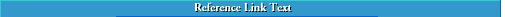## Numbers

Unless you are following a style guide that specifies otherwise, observe the following guidelines in using numbers.

• In general, use arabic numerals instead of words in scientific and technical writing for both cardinal and ordinal numbers:

• ``` 3 subroutines 6 braces 61 amino acids the 3rd subroutine the 6th brace the 61st amino acid ```

• In some scientific and technical styles, however, write out numbers between one and ten and two-word fractions that do not follow an integer:

• ``` three subroutines six braces 61 amino acids 1½ hours one-half hour ```

• If you need to begin a sentence with a number, spell it out. It is better, however, to reword the sentence, if possible.

• ```Thirty-two workstations were provided by the university.```

```The university provided 32 workstations.```

• If one number immediately follows another in a sentence, spell out the one that can be expressed in fewer words. Try, however, to express units of measure in arabic numerals. Another solution is to reword the sentence.

• ```We then prepared eight 20 ml samples.```

```We then prepared 8 samples of 20 ml each. ```

• Always spell out the pronoun one:

• ```In protecting the privacy of individuals, one must always consider all the possible ways data can be accessed and used.```

• Use numerals for dates, time of day, pages, figures, and notes:

• ```5 August 1994 1 p.m. page 43 Ref. 6 ```

``` Figure 43```

• Use arabic numerals followed by the percent sign, (%), to express percentages, except when the number begins a sentence:

• ```Only 3% of the systems crashed during the test period.```

```Three percent of the systems crashed during the test period. ```

#### British and American Formation of Numbers

• Form integers of two to four digits without any punctuation or spacing:

• ```21 412 ```

``` 8024```

• Use the period (.) to indicate the decimal point and use an initial zero (0) for numbers less than 1.0:

``` 31.3 1.41 ```

``` 0.414```

• Form numbers of five digits or more by using a comma (,) to mark off groups of three digits, starting at the decimal point:

• ``` 10,000 12,341 12,341.34 ```

``` 12,432,421```

#### European and International Standards for Formation of Numbers

• Form integers of two to four digits without any punctuation or spacing:

• ```21 412 ```

``` 8024```

• Use the comma (,) to indicate the decimal point and use an initial zero (0) for numbers less than 1,0:

• ```31,3 1,41 ```

``` 0,414```

• Form numbers of five digits or more by using a period (.) to mark off groups of three digits, starting at the decimal point:

• ```10.000 12.341 12.341,34 ```

``` 12.432.421```

#### General Guidelines for Using Numbers in Nontechnical Prose

When using numbers in nonscientific text, spell out numbers less than one hundred or numbers of any size that begin a sentence. In addition, spell out round numbers of any size and use arabic numerals followed by the terms million and billion to express numbers larger than one million.

```seventy-three hours 173 hours nine hundred thousand two billion 2.3 million ```

``` 146 billion```## Numbers ##